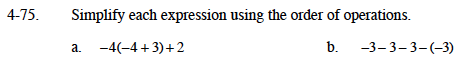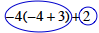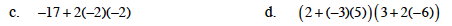### Home > MC1 > Chapter 4 > Lesson 4.2.3 > Problem4-75

4-75.
1. . Simplify each expression using the order of operations. Homework Help ✎

1. −4( −4 + 3) + 2

2. −3 − 3 − 3 − ( −3)

3. −17 + 2( −2)( −2)

4. (2 + ( −3(5))(3+2( −6))Circling terms should help with this one.−6−9

Simplify what's inside each set of large parentheses first.